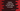# Learn Python numpy clip method with examples## numpy.clip method to limit the values in an array in Python:

numpy provides a method called clip to limit the values in an array. We can provide an interval, i.e. a maximum value and another minimum value, and if any value is not in this range, it is clipped to the interval edges.

In this post, we will learn the definition of clip and examples to learn how to use it.

### Definition of numpy.clip:

The numpy.clip method is defined as like below:

``numpy.clip(arr, min, max, out=None, **kwargs)``

Here,

• arr is an array holding the elements to clip.
• min and max are the edges for the clipping. min is the lower value and max is the upper value. Any value in the array arr smaller than min becomes min and any value bigger than max becomes max. Only one of these values may be None.
• out is an optional value, it can be a ndarray. The result is stored in this array. We can also provide the input array for in-place clipping.
• **kwargs are other keyword-only arguments.

It returns the clipped array. All elements which are smaller than min are replaced by min and all elements which are bigger than max are replaced by max in the clipped array.

Let’s try this method with different examples:

### Example 1: numpy.clip with an one-dimensional array:

Let’s try numpy.clip with a 1-D array:

``````import numpy as np

arr = np.array([1, 2, 3, 4, 5, 6, 7, 8, 9])

out_arr = np.clip(arr, 3, 7)

print(f'Given array: {arr}')
print(f'Final array: {out_arr}')``````

It will print:

``````Given array: [1 2 3 4 5 6 7 8 9]
Final array: [3 3 3 4 5 6 7 7 7]``````

As you can see, all elements which are smaller than 3 are changed to 3 and which are bigger than 7 are changed to 7.

### Example 2: numpy.clip with min > max:

This method doesn’t check if min is smaller than max or not. For example:

``````import numpy as np

arr = np.array([1, 2, 3, 4, 5, 6, 7, 8, 9])

out_arr = np.clip(arr, 7, 3)

print(f'Given array: {arr}')
print(f'Final array: {out_arr}')``````

It will run and it will change all values to 3.

``````Given array: [1 2 3 4 5 6 7 8 9]
Final array: [3 3 3 3 3 3 3 3 3]``````

### Example 3: Inplace replacement using numpy.clip:

If we pass the array to out, it will do the replacement in place, i.e. it will modify the original array:

``````import numpy as np

arr = np.array([1, 2, 3, 4, 5, 6, 7, 8, 9])

print(f'Given array: {arr}')

np.clip(arr, 3, 7, out=arr)

print(f'Final array: {arr}')``````

It will modify arr and it will print the below output:

``````Given array: [1 2 3 4 5 6 7 8 9]
Final array: [3 3 3 4 5 6 7 7 7]``````

### Example 4: Using only one parameter:

We can pass None for one of max or min and a value for the other:

``````import numpy as np

arr = np.array([1, 2, 3, 4, 5, 6, 7, 8, 9])

print(f'Given array: {arr}')

np.clip(arr, a_min=3, a_max=None, out=arr)

print(f'Final array: {arr}')``````

It will give:

``````Given array: [1 2 3 4 5 6 7 8 9]
Final array: [3 3 3 4 5 6 7 8 9]``````

If we don’t pass any of the min or max values, it will throw a ValueError.

``````raise ValueError("One of max or min must be given")
ValueError: One of max or min must be given``````